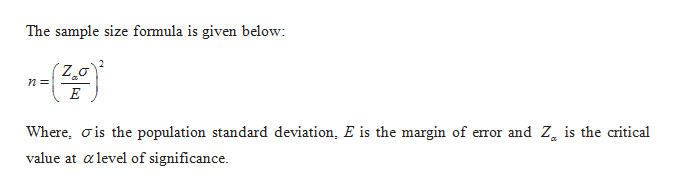# A survey is being planned to determine the mean amount of time corporation executives watch television. A pilot survey indicated that the mean time per week is 20 hours, with a standard deviation of 5.5 hours. It is desired to estimate the mean viewing time within 30 minutes. The 95% level of confidence is to be used. (Use  z Distribution Table.)How many executives should be surveyed? (Round your z-score to 2 decimal places and round up your final answer to the next whole number.)

Question
4 views
 A survey is being planned to determine the mean amount of time corporation executives watch television. A pilot survey indicated that the mean time per week is 20 hours, with a standard deviation of 5.5 hours. It is desired to estimate the mean viewing time within 30 minutes. The 95% level of confidence is to be used. (Use  z Distribution Table.) How many executives should be surveyed? (Round your z-score to 2 decimal places and round up your final answer to the next whole number.)
check_circle

Step 1

The sample size formula is given below:help_outlineImage TranscriptioncloseThe sample size formula is given below: (Zoa Where, is the population standard deviation, E is the margin of emror and Zg is the critical value at alevel of significance. fullscreen
Step 2

It is given that the mean time per week is 20 hours and a standard deviation of 5.5 hours.

Step 3

It is desired to estimate the mean time within 30 minutes. The significance level is 0.05.

Conve...

### Want to see the full answer?

See Solution

#### Want to see this answer and more?

Solutions are written by subject experts who are available 24/7. Questions are typically answered within 1 hour.*

See Solution
*Response times may vary by subject and question.
Tagged in

### Probability